StatLect

# Convolutions

In probability theory, convolution is a mathematical operation that allows to derive the distribution of a sum of two random variables from the distributions of the two summands.

In the case of discrete random variables, the convolution is obtained by summing a series of products of the probability mass functions (pmfs) of the two variables.

In the case of continuous random variables, it is obtained by integrating the product of their probability density functions (pdfs).## Convolution of probability mass functions

Letbe a discrete random variable with supportand probability mass function.

Letbe another discrete random variable, independent of, with supportand probability mass function.

The probability mass functionof the sumcan be derived by using one of the following two formulae: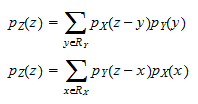These two summations are called convolutions.

Example Letbe a discrete variable with supportand pmf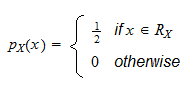Letbe another discrete variable, independent of, with supportand pmfTheir sumhas supportThe pmf ofneeds to be calculated for every. For, we have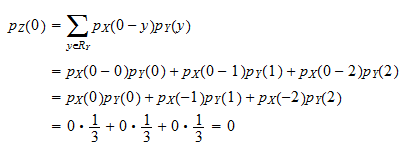For, we get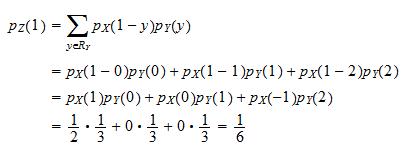For, the pmf is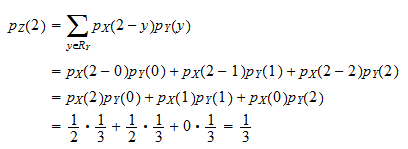And so on, until we obtain the value offor all.

## Convolution of probability density functions

Ifandare continuous, independent, and have probability density functionsandrespectively, the convolution formulae become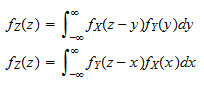Example Letbe a continuous variable with supportand pdf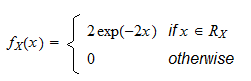that is,has an exponential distribution. Letbe another continuous variable, independent of, with supportand pdfthat is,has a uniform distribution. DefineThe support ofisWhen, the pdf ofis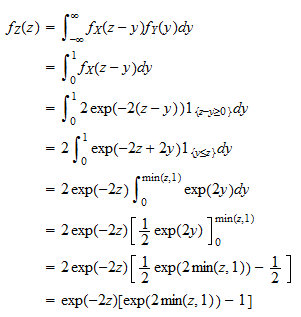Therefore, the probability density function ofis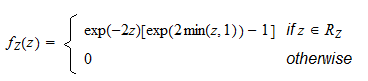## More details

A more detailed explanation of the concept of convolution and the proofs of the two convolution formulae can be found in the lecture entitled Sums of independent random variables.